# Line and Angle Relationships Chapter 1 Line and

• Slides: 18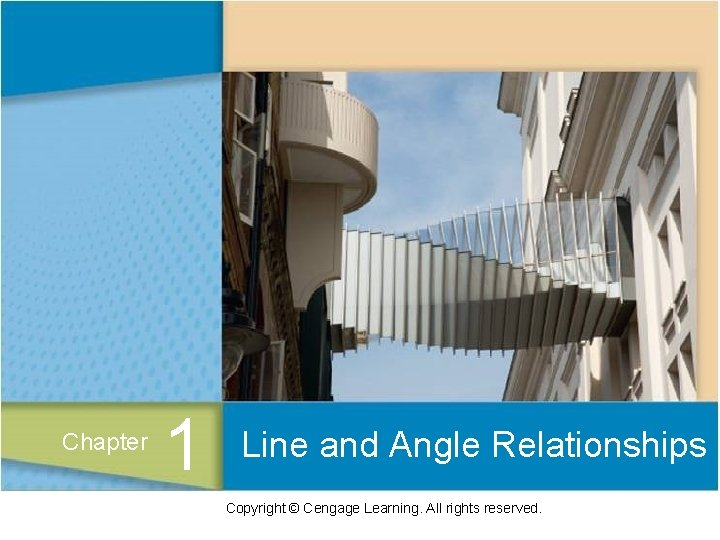STATEMENTS 3Statements Definition A statement is a set of words and/or symbols that collectively make a claim that can be classified as true or false. 4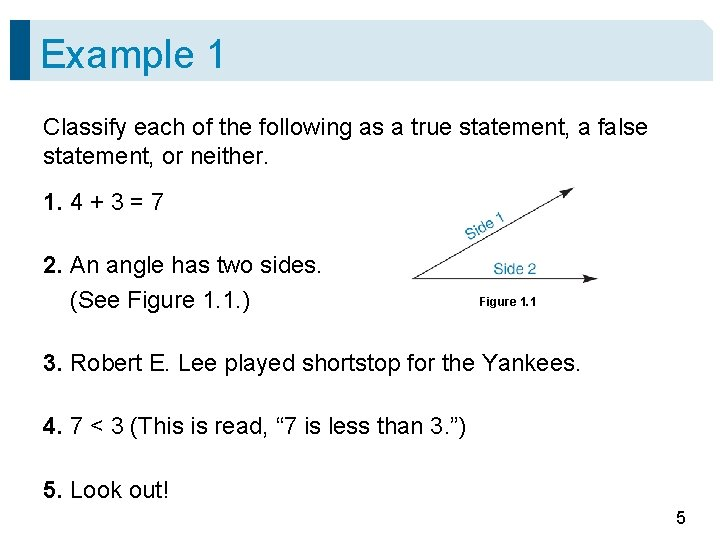Example 1 Classify each of the following as a true statement, a false statement, or neither. 1. 4 + 3 = 7 2. An angle has two sides. (See Figure 1. 1. ) Figure 1. 1 3. Robert E. Lee played shortstop for the Yankees. 4. 7 < 3 (This is read, “ 7 is less than 3. ”) 5. Look out! 5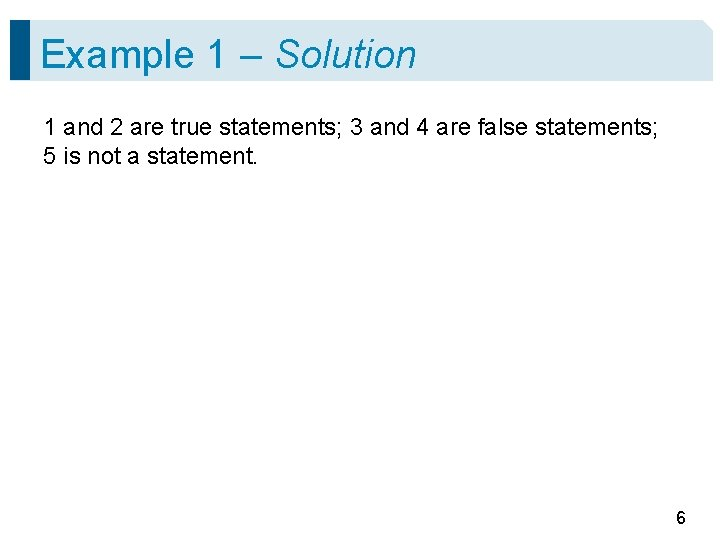Example 1 – Solution 1 and 2 are true statements; 3 and 4 are false statements; 5 is not a statement. 6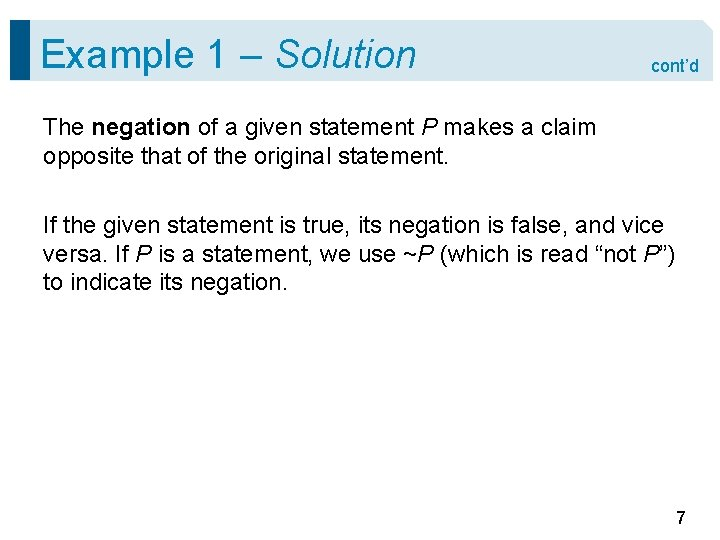Example 1 – Solution cont’d The negation of a given statement P makes a claim opposite that of the original statement. If the given statement is true, its negation is false, and vice versa. If P is a statement, we use ~P (which is read “not P”) to indicate its negation. 7Statements A compound statement is formed by combining other statements used as “building blocks. ” In such cases, we may use letters such as P and Q to represent simple statements. For example, the letter P may refer to the statement “ 4 + 3 = 7, ” and the letter Q to the statement “Babe Ruth was a U. S. president. ” 8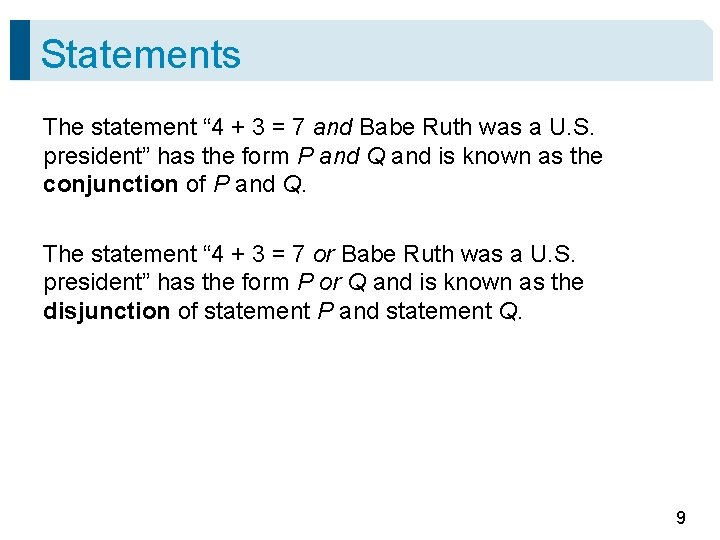Statements The statement “ 4 + 3 = 7 and Babe Ruth was a U. S. president” has the form P and Q and is known as the conjunction of P and Q. The statement “ 4 + 3 = 7 or Babe Ruth was a U. S. president” has the form P or Q and is known as the disjunction of statement P and statement Q. 9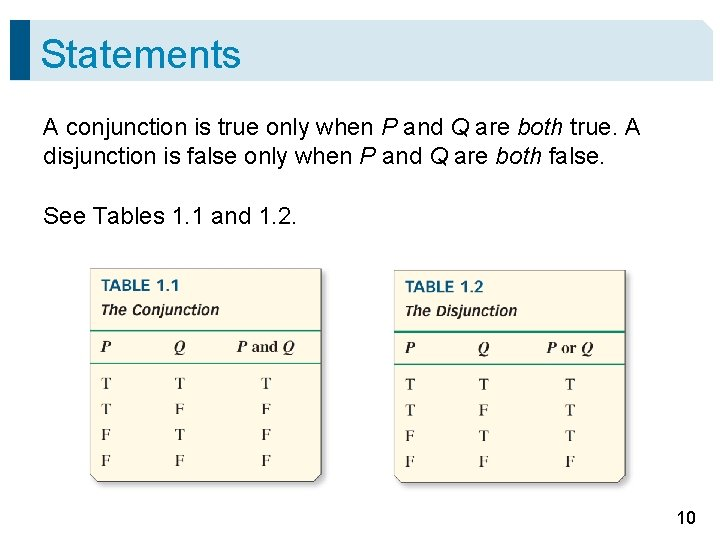Statements A conjunction is true only when P and Q are both true. A disjunction is false only when P and Q are both false. See Tables 1. 1 and 1. 2. 10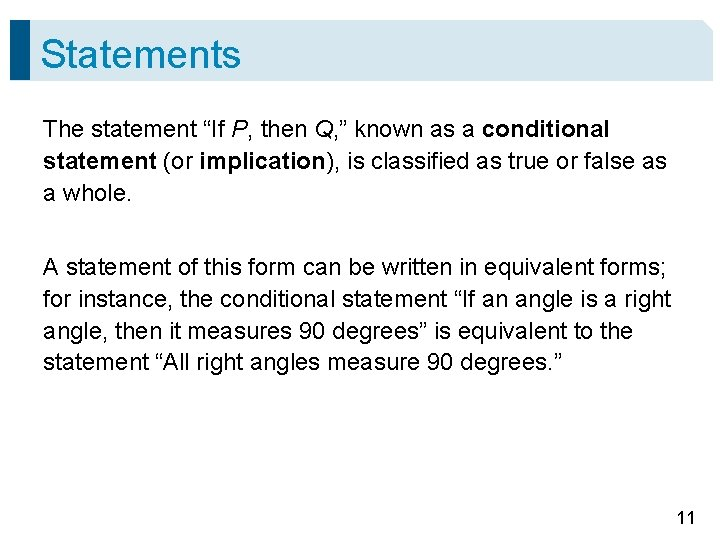Statements The statement “If P, then Q, ” known as a conditional statement (or implication), is classified as true or false as a whole. A statement of this form can be written in equivalent forms; for instance, the conditional statement “If an angle is a right angle, then it measures 90 degrees” is equivalent to the statement “All right angles measure 90 degrees. ” 11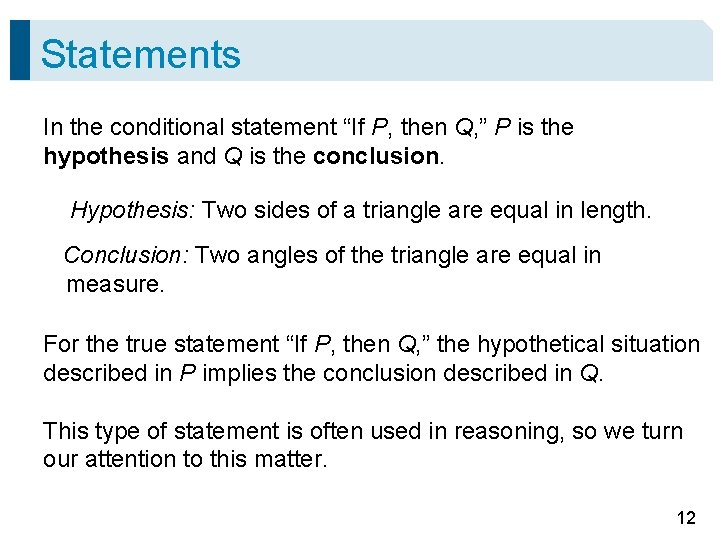Statements In the conditional statement “If P, then Q, ” P is the hypothesis and Q is the conclusion. Hypothesis: Two sides of a triangle are equal in length. Conclusion: Two angles of the triangle are equal in measure. For the true statement “If P, then Q, ” the hypothetical situation described in P implies the conclusion described in Q. This type of statement is often used in reasoning, so we turn our attention to this matter. 12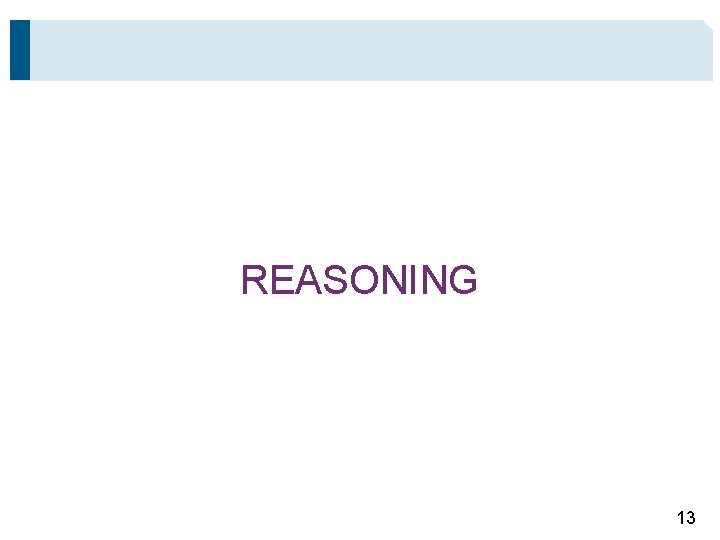REASONING 13Transformations Deduction Definition Deduction is the type of reasoning in which the knowledge and acceptance of selected assumptions guarantee the truth of a particular conclusion. In Example 8, we will illustrate a valid argument, a form of deductive reasoning used frequently in the development of geometry. 14Reasoning In this form, at least two statements are treated as facts; these assumptions are called the premises of the argument. On the basis of the premises, a particular conclusion must follow. This form of deduction is called the Law of Detachment. 15Example 8 If you accept the following statements 1 and 2 as true, what must you conclude? 1. If a student plays on the Rockville High School boys’ varsity basketball team, then he is a talented athlete. 2. Todd plays on the Rockville High School boys’ varsity basketball team. Conclusion Todd is a talented athlete. 16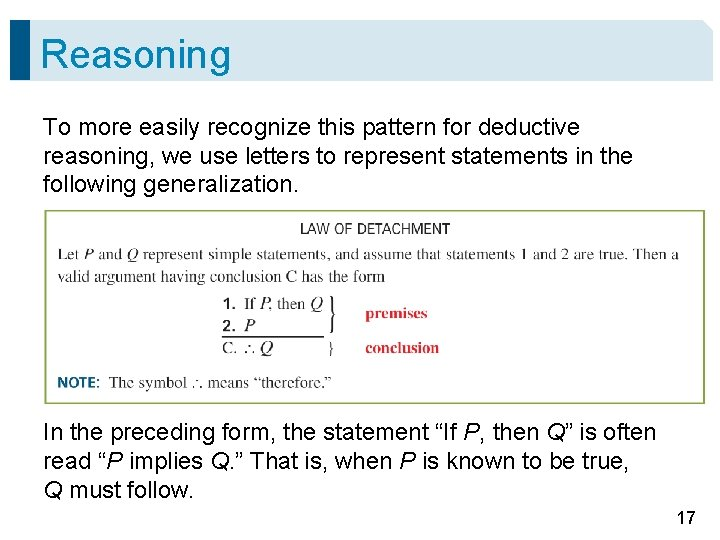Reasoning To more easily recognize this pattern for deductive reasoning, we use letters to represent statements in the following generalization. In the preceding form, the statement “If P, then Q” is often read “P implies Q. ” That is, when P is known to be true, Q must follow. 17Reasoning We will use deductive reasoning throughout our work in geometry. For example, suppose that you know these two facts: 1. If an angle is a right angle, then it measures 90°. 2. Angle A is a right angle. Because the form found in statements 1 and 2 matches the form of the valid argument, you may draw the following conclusion. C. Angle A measures 90°. 18